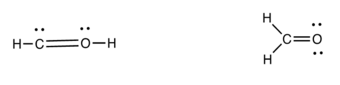# Problem: The formaldehyde molecule, which has the formula CH 2O, has two possible structures for which we can write a correct Lewis dot structures. Using formal charge, determine the correct structure.  Be sure to show how you compute the formal charge on each atom.

🤓 Based on our data, we think this question is relevant for Professor Richert's class at ASU.

###### Problem Details

The formaldehyde molecule, which has the formula CH 2O, has two possible structures for which we can write a correct Lewis dot structures. Using formal charge, determine the correct structure.  Be sure to show how you compute the formal charge on each atom.What scientific concept do you need to know in order to solve this problem?

Our tutors have indicated that to solve this problem you will need to apply the Formal Charge concept. You can view video lessons to learn Formal Charge. Or if you need more Formal Charge practice, you can also practice Formal Charge practice problems.

What is the difficulty of this problem?

Our tutors rated the difficulty ofThe formaldehyde molecule, which has the formula CH 2O, has ...as medium difficulty.

How long does this problem take to solve?

Our expert Chemistry tutor, Jules took 2 minutes and 21 seconds to solve this problem. You can follow their steps in the video explanation above.

What professor is this problem relevant for?

Based on our data, we think this problem is relevant for Professor Richert's class at ASU.